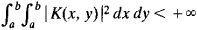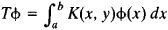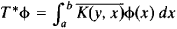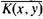Also found in: Dictionary, Wikipedia.

[′aj‚ȯint ′äp·ə‚rād·ər]
(mathematics)
An operator B such that the inner products (Ax,y) and (x,By) are equal for a given operator A and for all elements x and y of a Hilbert space. Also known as associate operator; Hermitian conjugate operator.

a concept of operator theory. Two bounded linear operators T and T* on a Hilbert space H are said to be adjoint if, for all vectors x and y in H,

(Tx, y) = (x, T*y)

For example, ifthen the adjoint of the operatoriswhereis the complex conjugate of the function K(x, y).

If T is not bounded and if its domain of definition Dm is everywhere dense (seeDENSE AND NONDENSE SETS), then the adjoint of T is defined on the set of vectors y for which a vector y* can be found such that the equality (Tx, y) = (x, y*) holds for all xDm. It is assumed here that T*y = y*.

The concept of adjoint operator can be extended to operators in other spaces.

Site: Follow: Share:
Open / Close